Next: 2.4.2 Tunnel Rate Up: 2.4 Tunneling Previous: 2.4 Tunneling

## 2.4.1 Transmission Probability

A wave packet with a probability amplitude B1 hits a potential barrier. Part of the wave will be reflected and the rest will be transmitted through the barrier. The probability amplitude of the transmitted part is denoted by B3. The transmission probability |T|2 is defined as the square of the ratio between transmitted and incoming probability amplitudes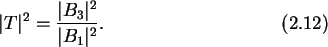The transmission probability can be derived from Schrödinger's equation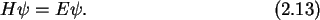For a rectangular potential barrier with width d and height E0 it is (see Appendix C.3 for a derivation)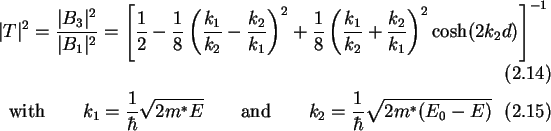For a typical  strength parameter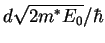of 30 the transmission probability is shown in Fig. 2.4. The strength parameter allows a classification of tunnel barriers according to their  opaqueness.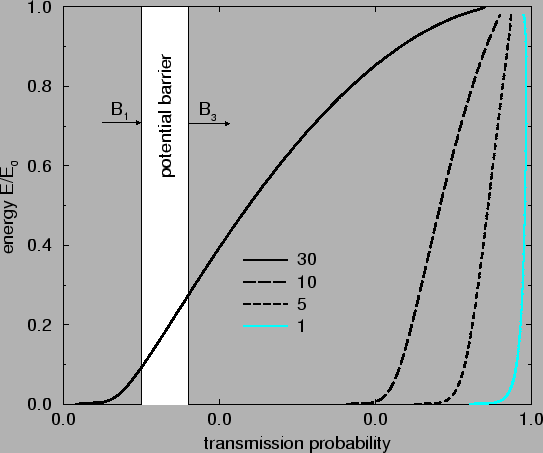A strength parameter of 1 is typical of the barriers present in most ohmic contacts to semiconductors, a value of 5 is typical for the ionization of an atom by an electric field and a value of 30 is characteristic for the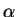-decay from a radioactive nucleus .Next: 2.4.2 Tunnel Rate Up: 2.4 Tunneling Previous: 2.4 Tunneling

Christoph Wasshuber